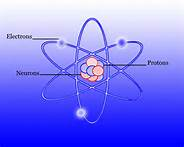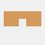# Chemistry - AtomsThere are three basic parts of an atom. They are the proton, neutron and the electron

The proton has a positive$(+)$ charge, the neutron has a neutral$(0)$ charge, and the electron has a negative$(-)$ charge

Amount

Protons

• The number of protons is simply the atomic number of the chemical in the periodic table

Electrons

• The number of electrons in an atom is the number of protons so that it can have a neutral charge

• But an atom may also lose or get electrons and that may cause it to be an ion

Neutrons

• You can get the number of neutrons in each atom here

• Or, you get the mass number of a chemical, that is the atomic weight rounded to the nearest ones, then subtract it by the number of protons

In example,

the atomic weight of carbon is $12.0107$, so the mass number is $12$

mass number = number of protons + number of neutrons

number of neutrons = mass number - number of protons

number of neutrons = $12-6$

number of neutrons = $6$ in a carbon atom

Properties

Protons

• It is in the centre of the nucleus of an atom

Neutrons

• It is also in the centre of the nucleus with the proton

Electrons

• The electron is special as it moves all around the nucleus because of the attraction of the protons. (It has a negative charge and proton has a positive charge, and they both will attract to each other like a magnet)

• Its mass is about $1000$ smaller than the protonNote by Daniel Lim
7 years, 4 months ago

This discussion board is a place to discuss our Daily Challenges and the math and science related to those challenges. Explanations are more than just a solution — they should explain the steps and thinking strategies that you used to obtain the solution. Comments should further the discussion of math and science.

When posting on Brilliant:

• Use the emojis to react to an explanation, whether you're congratulating a job well done , or just really confused .
• Ask specific questions about the challenge or the steps in somebody's explanation. Well-posed questions can add a lot to the discussion, but posting "I don't understand!" doesn't help anyone.
• Try to contribute something new to the discussion, whether it is an extension, generalization or other idea related to the challenge.
• Stay on topic — we're all here to learn more about math and science, not to hear about your favorite get-rich-quick scheme or current world events.

MarkdownAppears as
*italics* or _italics_ italics
**bold** or __bold__ bold
- bulleted- list
• bulleted
• list
1. numbered2. list
1. numbered
2. list
Note: you must add a full line of space before and after lists for them to show up correctly
paragraph 1paragraph 2

paragraph 1

paragraph 2

[example link](https://brilliant.org)example link
> This is a quote
This is a quote
    # I indented these lines
# 4 spaces, and now they show
# up as a code block.

print "hello world"
# I indented these lines
# 4 spaces, and now they show
# up as a code block.

print "hello world"
MathAppears as
Remember to wrap math in $$ ... $$ or $ ... $ to ensure proper formatting.
2 \times 3 $2 \times 3$
2^{34} $2^{34}$
a_{i-1} $a_{i-1}$
\frac{2}{3} $\frac{2}{3}$
\sqrt{2} $\sqrt{2}$
\sum_{i=1}^3 $\sum_{i=1}^3$
\sin \theta $\sin \theta$
\boxed{123} $\boxed{123}$

## Comments

Sort by:

Top Newest

Do add quantum no.s too

- 5 years, 7 months ago

Log in to reply

i just han ana exam about it :D

- 7 years, 4 months ago

Log in to reply

×

Problem Loading...

Note Loading...

Set Loading...